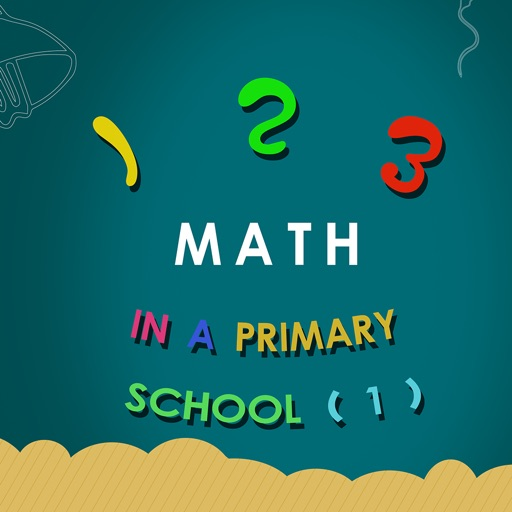## A Complete K-12 math curriculum, from counting to calculus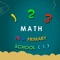# 123 math in a primary school

by 涛 陈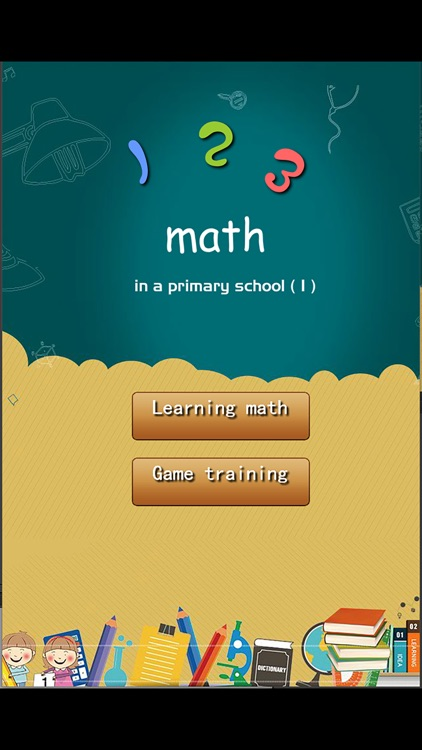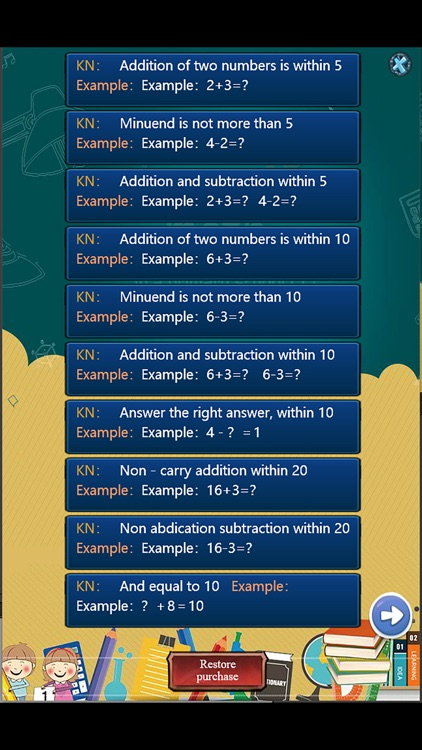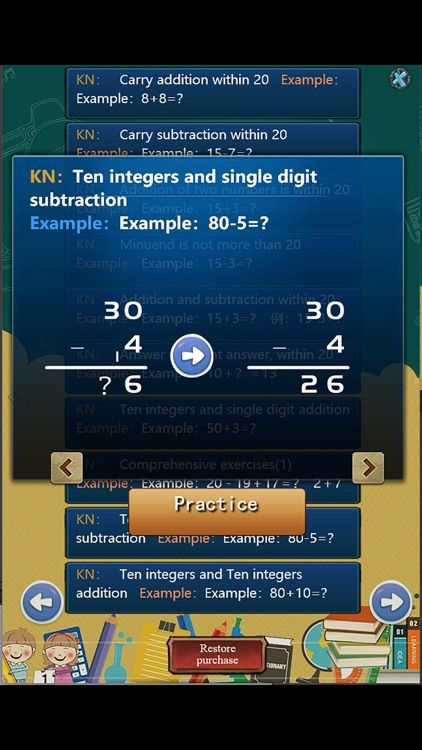A Complete K-12 math curriculum, from counting to calculus.takes the child through addition, subtraction, multiplication, division, and so upward until calculus,This is part of the first part.Version
1.9
Rating
NA
Size
13Mb
Genre
Education Travel
Last updated
July 13, 2022
Release date
August 11, 2018

### App Screenshots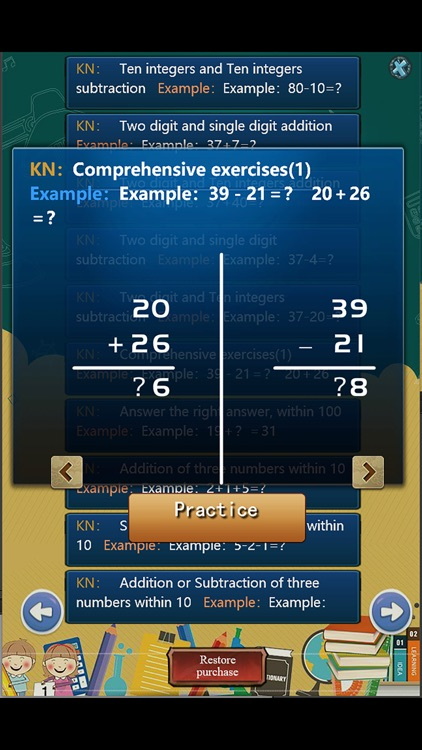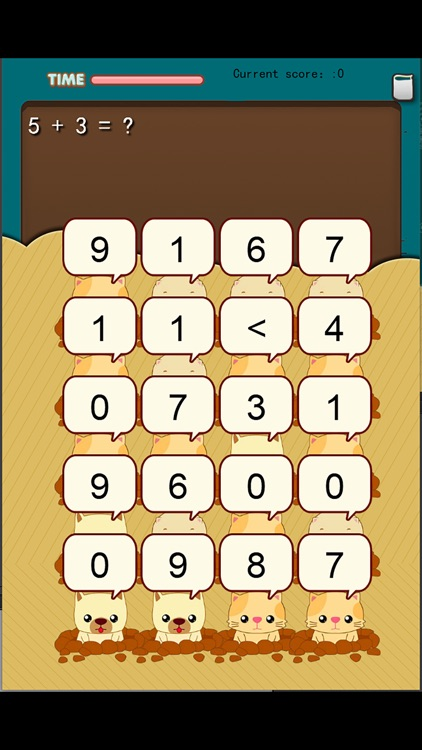### App Store Description

A Complete K-12 math curriculum, from counting to calculus.takes the child through addition, subtraction, multiplication, division, and so upward until calculus,This is part of the first part.
As you can see, this set of Arithmetic APP is organized in an orderly manner around the discipline of arithmetic itself.

This application includes:

Addition of two numbers is within 5

Minuend is not more than 5

Addition of two numbers is within 10

Minuend is not more than 10

Non - carry addition within 20

Non abdication subtraction within 20

And equal to 10

Carry subtraction within 20

Addition of two numbers is within 20

Minuend is not more than 20

Ten integers and single digit addition

Comprehensive exercises(1)

Ten integers and single digit subtraction

Ten integers and Ten integers addition

Ten integers and Ten integers subtraction

Two digit and single digit addition

Two digit and Ten integers addition

Two digit and single digit subtraction

Two digit and Ten integers subtraction

Comprehensive exercises(2)

Addition of three numbers within 10

Subtraction of three numbers within 10

Addition or Subtraction of three numbers within 10

Addition or Subtraction of two numbers within 10

Addition of three numbers within 20

Subtraction of three numbers within 20

Addition or Subtraction of three numbers within 20

Addition or Subtraction of three numbers within 20

Disclaimer:
AppAdvice does not own this application and only provides images and links contained in the iTunes Search API, to help our users find the best apps to download. If you are the developer of this app and would like your information removed, please send a request to takedown@appadvice.com and your information will be removed.